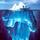## Send to a FriendAsked by gasman (11315) September 12th, 2011

I’ve worked with MS Office Excel spreadsheets before. I understand referencing other cells in a formula, specifying a range of cells, and using functions like SUM(ABOVE) OR SUM(LEFT). But I’m stumped on how to implement a couple of seemingly simple situations:

(1) Make a table where the first column consists of positive numbers and the second column is their square roots. Each cell in the 2nd column needs a formula that says, “Take the square root of the value in the cell to your left.” How would you write the formula? Do you have to enter a different formula for each row?

(2) Make a multiplication table for the integers 1–9 (like in 3rd grade) where the top row and leftmost column each contain values 1 through 9. Cell i9 would contain the number 81. How do you write a formula that says, “Take the first number in this row (i.e., the value of the first cell) & multiply by the first number in this column?”

or

#### Using Email

Separate multiple emails with commas.
We’ll only use these emails for this message.